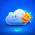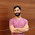in Japanese

## Introduction

In the previous page, I described the brief explanation on the theory of the EM Algorithm. In this page, I will use the implementation of the EM Algorithm the OpenCV provides to us to achieve a simple image segmentation. I will also relate the quantities the OpenCV computes to the expressions shown in the explanation.

## Expressions in Previous Page

To refer to some expressions used for the interpretation on the EM Algorithm, I describe them again.

The likelihood function to maximize is defined aswhere,is the normalized Gaussian,is the observed point,is the mean vector, andis the covariance matrix.indicates the weight of each Gaussian. Moreover, the following expressions also appeared during the process of the explanation.## Code

function main:
As I consider the RGB color space, an observed point corresponds to a pixel on an image, i.e.,.is the total number of the pixels.
1. the 3th-6th lines : Read an input image (image). Confirm the type of the image, and define varibles image_rows and image_cols.
2. the 10th-13th lines : Reshape image to make reshaped_image. Confirm its type and its number of rows and columns.
3. the 16th-20th lines : Convert the type of reshaped_image to make samples. Confirm its type and its number of rows and columns. The samples is one of some inputs passed to the EM Algorithm.
4. the 22th-23th lines : Create an instance of the EM Algorithm (cv::EM). Arguments passed to the constructor of the cv::EM are as follows:
• the first argument : the number of the Gaussians
• the second argument : the type of the covariance matrix
• the third argument : the condition to quit the algorithm
The first argument corresponds to. I used default values to the second and the third arguments. The default value of the second argument is EM::COV_MAT_DIAGONAL and it means a diagonal matrix with positive diagonal elements. The number of free parameters is D for each matrix, where D is the space dimension. In this sample code, D equals to 3.
5. the 26th-28th lines : Prepare a matrix for an output.
6. 31th line: Execute the EM Algorithm. The function train internally uses the K-means clustering to create initial parameters.
7. the 33th-35th lines : Confirm the type, the rows, and the columns of the output log_likelihoods. In each row the following quantity is stored.
8. the 37th-39th lines : Confirm the type, the rows, and the columns of the output labels. The labels has the following quantities:
(I modified the following statements. 2013/3/21)where,.is the probability of the observed point..
9. the 41th-44th lines : Confirm the type, the rows, and the columns of the output probs. The probs has the quantities. I will discuss the function observe_probs later.
10. the 46th-50th lines : Confirm the type, the rows, and the columns of the output means which has. As we consider the RGB space, the quantity is the 3D vector. I will discuss the function observe_labels_and_means later.
11. the 52th-56th lines : Confirm the type, the rows, and the columns of the output weights. In the output,is stored. I will discuss the function observe_weights later.
function observe_probs:
1. the 13th line : Confirm the following equation:2. the 16th line : Confirm the following equation:function observe_weights:
1. the 10th line : Confirm the following equation:function observe_labels_and_means:
This function replaces a label k assigned to a certain pixel by a corresponding mean vector.
1. the 4th line : Create a matrix in which a final result (rgb_image) is stored.
2. the 9th-11th lines : means is a cluster_number x 3 matrix, where 3 means the space dimension. In each row, the center coordinate (mean vector) of the Gaussian is stored. Apply the type conversion from double to unsigned char to the elements of means. Also, the number of the channels is converted to 3.
3. the 13th-18th lines : Replace the label by the corresponding (R,G,B) to make the final image rgb_image.

## Results

cluster_num=2
cluster_num=3

cluster_num=5
cluster_num=10
original image

## Development Environment

1. Mac OS X 10.8.2
2. Processor：3.06 GHz Intel Core 2 Duo
3. Memory：4GB
4. Xcode4.6 with Apple LLVM 4.2（C++ Language Dialect → GNU++11, C++ Standard Library → libc++）
5. boost-1.51.0 built by the Apple LLVM 4.1. See here
6. opencv-2.4.3 built by the Apple LLVM 4.2. See here.

## Reference

1. Pattern Recognition and Machine Learning, Christopher M. Bishop, Springer
2. OpenCV Document

#### 14 件のコメント:

1.Hi Seiya Kumada, thank you for your post. However, I have a strange problem with Expectation-Maximization. When I run your first code on Linux, it executes fine in 3 seconds. However, when I try the same code on Windows, it takes near 20 minutes to converge. Do you know what might be the problem?

1.Hi Victor Hugo.
Thank you for your comment.
After receiving it, I timed the calculations with cluster_numer = 10 on both Windows7 and Mac OSX. The results are as follows:
Mac OSX : about 280[sec]
My Windows machine has 4.0 GB RAM and Intel Core1.7GHz (core number 2).
So, I don't know what happened in your machine...

2.Hi Seiya,

I think I found my problem. I'm using a framework linked with several libraries. One of them is QT, which brought me problems before to manage screens. I think it might be causing problems to me again.

Thanks for your goodwill!

3.Hi Victor.
It's reassuring to know that.
I used Visual Studio 2010 to carry out the code on Windows7.
Since the compiler does not fully support C++11,
I modified the code a little.

Have a good time!

2.hi Seiya thank you very much for your post

1.You are welcome.

3.please don't lie to people. how can you say it's fully working with Mat rgb_image which doesn't exist of any further process?

4.Thank you very much for your clair explanation and your well written code. It was very helpful to me. The code works very good, i tried it in my computer for color/grayscale images

1.It's my pleasure.

5.Hi, thank you very much for the code and the explanation. It was exactly what I was looking for. Cheers!

1.I am happy to be of help.

6.Hi Seiya, thank you for your post.

Can I ask you a question?

I tried to copy and paste your code to my mac, (Macbook pro 13', 2015, El capitan)

and tried to run it with XCode, there is an error message with
cv::EM model {cluster_num};
(23rd line in your code)

Also, I found that this error makes some more errors.

How can I fix this problem?

Thanks. -From G.

7.OpenCV modified the structure of the class EM after I wrote this post. Please see the current API reference for more detail.

8.Thank you very much!